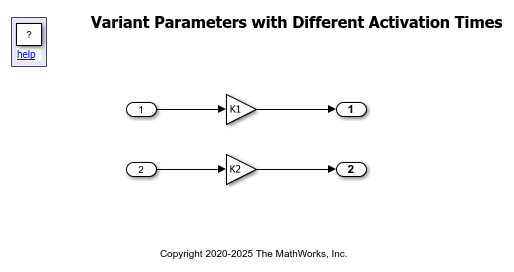# Change Active Values and Activation Time of Variant Parameters

This example shows how to change the active values and activation time of variant parameters in a Simulink® model.

In this example, you will learn how to:

• Change active values of variant parameters using variant condition expressions.

• Change the time of determining active values using variant activation time.

### Overview of Variant Parameters

Variant parameters can have multiple values. Each value of the variant parameter is associated with a variant control expression. During simulation, the value of a variant parameter associated with the condition that evaluates to `true` is the active value of the variant parameter. The value associated with the condition that evaluates to `false` is the inactive value of the parameter. To switch between the active values, change the value of the variant control variable. For more information, see Use Variant Parameters to Reuse Block Parameters with Different Values.

### Prerequisite

Before you start with this example, we recommend you complete Create a Simple Variant Parameter Model.

### Explore the Model

1. Open the model.

```open_system([matlabroot '/examples/simulink_variants/main/slexVariantParameters']) ```In this model, the Gain parameter of the Gain blocks are variant parameters that are specified as `Simulink.VariantVariable` objects `K1` and `K2`. The objects are defined in the `slexVariantParameterData.m` file.

2. Open the `slexVariantParameterData.m` file. The file is loaded in the PostLoadFcn callback of Modeling > Model Settings > Model Properties.

In the file, the objects `K1` and `K2` have multiple values associated with variant control expressions `V==1` and `V==2`. In addition to the value, you can specify attributes such as dimension, data type, and so on for each object using the Specification parameter of the object.

• Variant control variable: The value of the `Simulink.VariantControl` object `V` determines which values of `K1` and `K2` are active during simulation. If `V==1` evaluates to `true`, the values associated with `V==1` become active, and all the values associated with `V==2` become inactive. The active value of `K1` is assigned to the Gain parameter of the Gain1 block, and the active value of `K2` is assigned to the Gain parameter of the Gain2 block. Similarly, when `V==2` evaluates to `true`, all the values associated with `V==1` become inactive, and all the values associated with `V==2` become active. For more information on variant controls, see Introduction to Variant Controls.

• Variant activation time: Simulink can set active value of the variant parameter Gain at different stages of the simulation and code generation workflow. Based on the stage you specify as the variant activation time, Simulink determines if the generated code must contain only the active values or both active and inactive values of `K1` and `K2`. The variant activation time also determines whether to analyze all the values of `K1` and `K2` for incompatibilities in signal attributes.

In this example, the value of `V` is specified as `1`, and the variant activation time is specified as `update diagram`. When you simulate this model, `V==1` evaluates to `true`. The value of `K1` is set to `3.5` and the value of `K2` is set to `4.5`. Simulink determines the active values during update diagram. When you generate a code from this model, the code contains only the active values of `K1` and `K2`.

### Set Active Choice of Variant Parameters

The value of the variant control variable determines the active value of variant parameters during simulation. To switch the active values, change the value of variant control variable.

1. On the Simulink toolstrip, click Run. During simulation, `V==1` evaluates to `true`. As the values `3.5` and `4.5` are associated with `V==1`, these values are assigned to `K1` and `K2`, respectively. Subsequently, the values are assigned to the Gain parameters of the Gain blocks.

2. To change the value of the Gain parameters, change the value of `V` to `2`, then simulate the model again.

```V.Value = 2; ```

During simulation, all the values associated with `V==2` are assigned to `K1` and `K2`. Subsequently, the values are assigned to the Gain parameters of the Gain blocks.

### Set Time to Determine Active Value of Variant Parameters

Simulink can set active value of the variant parameters at different stages of the simulation and code generation workflow. Based on the stage you specify as the variant activation time, Simulink determines if the generated code must contain only the active values or both active and inactive values of variant parameters. The variant activation time also determines whether to analyze all the values the variant parameters for incompatibilities in signal attributes.

For example, to specify the activation time of `V` as `update diagram`, type this command in the MATLAB® Command Window. Then, simulate the model again.

```V.ActivationTime = 'update diagram'; ```

Simulink sets the active values of `K1` and `K2` during update diagram before the propagation of signal attributes. The signal attributes such as dimension and data type are not analyzed for consistency across the model. The generated code contains only the active value of `K1` and `K2`. For information on other activation times, see Activate Variant During Different Stages of Simulation and Code Generation Workflow.